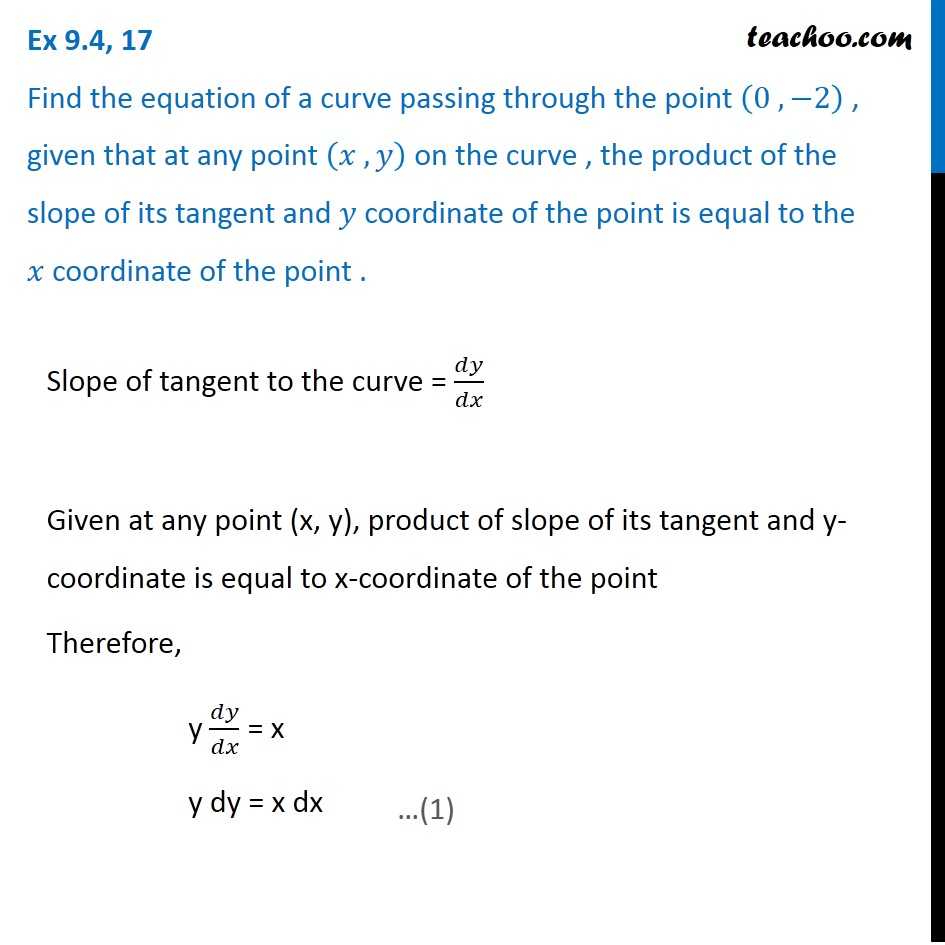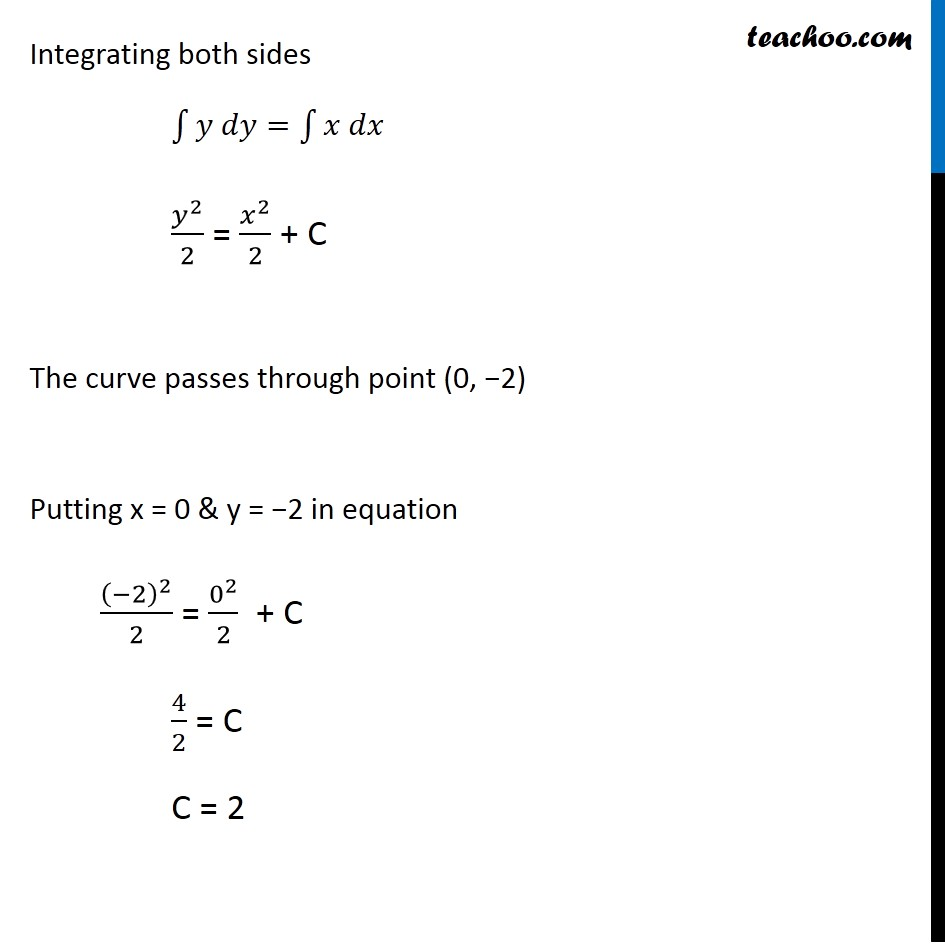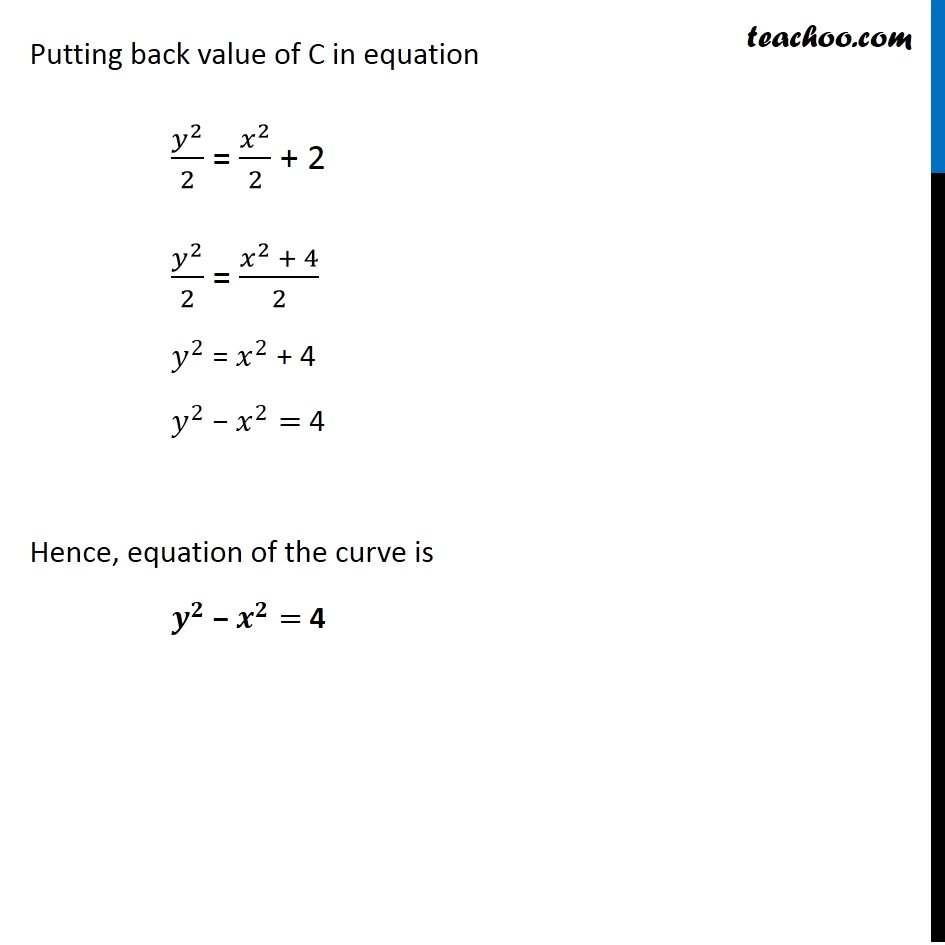1. Chapter 9 Class 12 Differential Equations
2. Concept wise
3. Variable separation - Statement given

Transcript

Ex 9.4, 17 Find the equation of a curve passing through the point (0 , −2) , given that at any point (𝑥 , 𝑦) on the curve , the product of the slope of its tangent and 𝑦 coordinate of the point is equal to the 𝑥 coordinate of the point . Slope of tangent to the curve = 𝑑𝑦/𝑑𝑥 Given at any point (x, y), product of slope of its tangent and y-coordinate is equal to x-coordinate of the point Therefore, y 𝑑𝑦/𝑑𝑥 = x y dy = x dx …(1) Integrating both sides ∫1▒〖𝑦 𝑑𝑦=∫1▒〖𝑥 𝑑𝑥 〗 〗 𝑦^2/2 = 𝑥^2/2 + C The curve passes through point (0, −2) Putting x = 0 & y = −2 in equation (−2)^2/2 = 0^2/2 + C 4/2 = C C = 2 Putting back value of C in equation 𝑦^2/2 = 𝑥^2/2 + 2 𝑦^2/2 = (𝑥^2 + 4)/2 𝑦^2 = 𝑥^2 + 4 𝑦^2 − 𝑥^2= 4 Hence, equation of the curve is 𝒚^𝟐 − 𝒙^𝟐= 4

Variable separation - Statement given